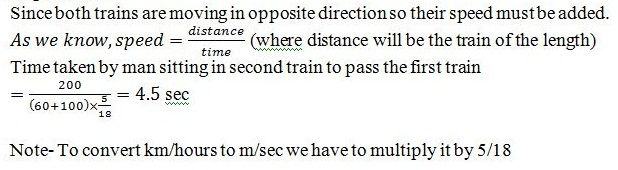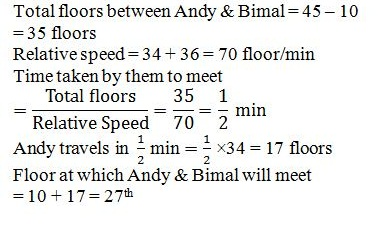# gkrecall

We brings you daily Current affairs, daily Current Affairs Quiz, weekly Current Affairs, weekly Current Affairs Quiz to enhance your preparation for upcoming exams. We also provide articles related to quant, reasoning, English, ssc etc .

## Breaking

QUANT PRACTICE SET 36 FOR SBI PO PRELIM 2020

SBI Clerk and SBI PO Prelims exam are going to be held in the upcoming months. We have already provided you with the PDFs of many topics of Quantitative Aptitude like Simplification/Approximation, Number Series,datainterpretation.important Arithmetic questions to prepare for SBI Clerk and PO Pre exam. Practicing these questions will help you to know about the level of the questions. To increase your speed and accuracy, enhance your calculations.

QNO 1: Car A leaves the city at 5pm and is driven at a speed of 30km/hr. 3hrs later another car B leaves the city in the same direction as car A. In how much time will car B be 12kms ahead of car A if the speed of car B is 50km/hr?
A) 5hrs
B) 4 hrs 12 mins
C) 8hrs
D) 5 hrs 6 mins
E) 12hrs
QNO 2: Two train starts at the same time from Delhi and Agra and proceed towards each other at the rate of 40km/hr and 37 1/2km/hr. When they meet it is found that one train has traveled 200km more than the other train. What is the distance between Delhi and Agra?
A) 6200km
B) 5000km
C) 4200km
D) 4800km
E) 6000km
QNO3: A man walks from A to B and cycles from B to A, a distance of 37.5 km in all spending 2 hours and 40 minutes. He would have taken 2/3rd hour less had he chosen to cycle the entire distance of 37.5 km. what would have been the time taken by him If he had chosen to walk both the ways?
a) 3 hours 30 min
b) 3 hours 20 min
c) 3 hours 12 min
d) 3 hours 25 min
e) None of these
QNO4:  Two persons start from the opposite ends of a 90 km straight track and run to and from between the two ends. The speed of first person is 30 m/s and the speed of other is 125/6 m/s. They continue their motion for 10 hours. How many times they pass each other?
a) 10
b) 9
c) 12
d) 15

QNO5: Raghav drives his truck very fast at 360 kmph. Moving ahead for some hours he finds some problem in headlights of the truck. So he takes 20 seconds in changing in the bulb of the headlight by stopping the truck. Mean while he notices that 2nd truck which was 400 m back is now 200 m ahead of his truck. What is the speed of 2nd truck?
a) 100 kmph
b) 92 kmph
c) 108 kmph
d) 300 kmph
e) None of these

QNO6: Towns A and B are 225 km apart. Two cars P and Q travel towards each other from towns A and B respectively and meet after 3 hours. If the speed of P be 1/2 of its original speed and Q be 2/3 of its original speed, they would have met after 5 hours. Find the speed of the faster car.
A) 50 km/hr
B) 40 km/hr
C) 45 km/hr
D) 30 km/hr
E) 60 km/hr

QNO7: Two trains 200 m and 160 m long, run at the rate of 60 km/h and 100 km/h respectively on parallel rails. How long will it take a man sitting in the second train to pass the first train if they run in the opposite direction?
1) 4.4 seconds
2) 4.5 seconds
3) 3.24 seconds
4) 4 seconds
5) None of these
QNO8: Andy lives on 10th floor and Bimal lives on 45th floor. Andy goes up at a rate of 34 floors per minute and Bimal comes down at a rate of 36 floors per minute. At which floor they will meet?
1) 27
2) 26
3) 18
4) 32
5) None of these
QNO9: Two trains are travelling in same direction with 60 km/hr and 75 km/hr respectively. The faster train crosses a man sitting in the slower train in 30 sec. find the length of faster train.
a) 100 meter
b) 125 meter
c) 140 meter
d) 150 meter
e) None of these
QNO10: A train running at 45 km/hr takes 36 sec to pass a platform. Next, the train takes 12 sec to pass a man walking at the speed of 15 km/hr in the same direction. Find the length of the platform.
a) 250m
b) 300m
c) 350m
d) 400m
e) None of these
QNO11: Two stations A and B are 150 km apart from each other. One train starts from A at 6 AM at a speed of 30 km/hr and travels towards B. Another train starts from station B at 7 AM at a speed of 20 km/hr. At what time they will meet.
a) 9:34 AM
b) 10:34 AM
c) 8:34 AM
d) 7:34 AM
e) None of these
QNO12:  Shivam travels 20% distance of the total journey by car and 50% of the remaining by train and taxi in the respective ratio of 5:3 and the remaining distance he covers on feet. If the sum of the distance which he travels by car and by Taxi is 126 km, then find the total distance which Shivam travels during his journey?
1)      A 360 km
2)      B 640 km
3)      C 420 km
4)      D 400 km
5)      E 210 km
QNO13: A beats B by 15 sec in a 200 m race, B beats C by 25 sec in a 500 m race, C beats D by 32 sec in  800 m race and D beats E by 35 sec in a 1 km race. What must be the speed of A in order to beat E by 800 m in a 2 km race?
1)    2.5 M/S
2)    3.33 M/S
3)    5 M/S
4)    6.66 M/S
5)    NONE OF THESE
QNO14: The distance between Kanpur and Bangalore by flight is 1800 km. An aircraft was slowed down due to bad weather. The time of journey of Bangalore from Kanpur is increased by 30 minutes and the average speed for the journey was reduced by 300 km/h. What is the usual duration of the flight if there is no bad weather?
1)    3/2 hr
2)    4/5hr
3)    6/5hr
4)    2/3hr
5)    NONE OF THESE
QNO15: A bus is running with a speed of 30 km/h on the road beside a railway track. The bus is 260 metres ahead of the engine of a 440 metres long train running with the speed 156 km/h in the same direction. In how much time will the train pass the bus completely?
1)    20 sec
2)    40 sec
3)    35 sec
4)    25 sec
5)    None of these

SOLUTIONS:

Car A travels 3hrs. 3*30=90km
Difference between speeds 50-30=20km/hr
T=D/S ===>102/20=5 hrs 6 mins

Speed ratio 40:37 1/2==>40: 75/2==>80:75 ie 16:15
ratio diff  between speed is 1[16-15] 1 ===> 200 (more distance)
[16+15]31 ===>?
31*200=6200km.

He takes 40 minutes less to cycle than to walk. Thus, on the whole he takes 40 more.
Required time = 3 hours 20 minutes
The speeds of two persons is 108 kmph and 75 kmph.
The first person covers (108*10)=1080 km in 10 hours and thus he makes (1080/90)=12 rounds.
Thus, he will pass over another person 12 times in any one of the direction.
Speed of 2nd truck = [(400 + 200)/20] * [18/5] kmph  = 108 kmph
Let speeds be x km/hr and y km/hr
So 225/(x+y) = 3
And 225/(x/2 + 2y/3) = 5
Solve, x = 30, y = 45
Explanation :Explanation :Explanation :
L = 15*5/18*30 = 125 meter

Explanation :
Let ‘T’ and ‘P’ are the length of train and platform respectively
T = 12*30*5/18 = 100 meter
P + 100 = 45*5/18*36
P= 350

Explanation :
Distance travel by first train in one hour = 30, now the distance remains 120 km only.
x/30 = (120 – x)/20, so we get x = 72 km
Now, time = (30 + 72)/30 = 3hrs and 24minutes i.e. 9: 24 am

12.A
Let total distance = 100x

Distance covered by car = 20% of 100x = 20x

 Distance covered by Train = 5 × 50% of (100x – 20x) = 5 × 40 x = 25x 5 + 3 8

 Distance covered by Taxi = 3 × 50% of (100x – 20x) = 3 × 40 x = 15x 5 + 3 8

Distance covered on feet = 100x – 20x – 25x – 15x = 40x

Total distance covered by Car and Taxi = 20x + 15x = 35x = 126 km (Given)

 Length of journey = 100 × 126 = 360 km 35

Hence, option (A) is correct.

13.B
A beats B by 15 sec means A reaches the destination 15 sec ahead of B or B reaches 15 sec later than B.  Let all of them compete in a 2 km  or 2000 m race, now we can compare them as follows:
A beats B by 150 sec, B beats C by 100 sec, C beats D by 80 sec, D beats E by 70 sec, ⇒ E would finish the race 400 sec after A.
Now, if A has to beat E by 800 m then we can say that E will cover the remaining 800m in 400sec, and cover 2000m in 1000 sec. A has to reach 400s earlier i.e in 600 sec,
 so A’s speed = 2000 = 3.33 m/s. 600

Hence, option (B) is correct.

14.A
Let the usual duration of the flight be X hours

 Usual speed of the flight = 1800 X

 Then, 1800 – 1800 = 300 X X + 1 2

 ⇒ 1800 – 3600 = 300 X 2X + 1

⇒ X(2X + 1) = 6

⇒ 2X2 + X – 6 = 0
 ⇒ X = 3 hours 2

Hence, option (A) is correct.

15.A
Relative speed of the train with respect to the bus = 156 – 30 = 126km/h

 = 126 × 5 18

= 35m/s

Distance = 440 + 260 = 700 metre

 Required time = 700 = 20 seconds 35

Hence, option (A) is correct.